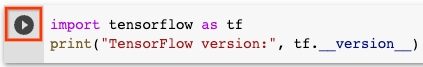# TensorFlow 2 quickstart for beginners

This short introduction uses Keras to:

2. Build a neural network machine learning model that classifies images.
3. Train this neural network.
4. Evaluate the accuracy of the model.

This tutorial is a Google Colaboratory notebook. Python programs are run directly in the browser—a great way to learn and use TensorFlow. To follow this tutorial, run the notebook in Google Colab by clicking the button at the top of this page.

1. In Colab, connect to a Python runtime: At the top-right of the menu bar, select CONNECT.
2. To run all the code in the notebook, select Runtime > Run all. To run the code cells one at a time, hover over each cell and select the Run cell icon.## Set up TensorFlow

Import TensorFlow into your program to get started:

``````import tensorflow as tf
print("TensorFlow version:", tf.__version__)
``````
```TensorFlow version: 2.13.0
```

If you are following along in your own development environment, rather than Colab, see the install guide for setting up TensorFlow for development.

Load and prepare the MNIST dataset. The pixel values of the images range from 0 through 255. Scale these values to a range of 0 to 1 by dividing the values by `255.0`. This also converts the sample data from integers to floating-point numbers:

``````mnist = tf.keras.datasets.mnist

(x_train, y_train), (x_test, y_test) = mnist.load_data()
x_train, x_test = x_train / 255.0, x_test / 255.0
``````

## Build a machine learning model

Build a `tf.keras.Sequential` model:

``````model = tf.keras.models.Sequential([
tf.keras.layers.Flatten(input_shape=(28, 28)),
tf.keras.layers.Dense(128, activation='relu'),
tf.keras.layers.Dropout(0.2),
tf.keras.layers.Dense(10)
])
``````

`Sequential` is useful for stacking layers where each layer has one input tensor and one output tensor. Layers are functions with a known mathematical structure that can be reused and have trainable variables. Most TensorFlow models are composed of layers. This model uses the `Flatten`, `Dense`, and `Dropout` layers.

For each example, the model returns a vector of logits or log-odds scores, one for each class.

``````predictions = model(x_train[:1]).numpy()
predictions
``````
```array([[ 0.08467684, -0.6962797 ,  0.18567504, -0.2014905 ,  0.6376542 ,
0.5915777 , -0.45832562, -0.23375851,  0.11700233, -0.03049438]],
dtype=float32)
```

The `tf.nn.softmax` function converts these logits to probabilities for each class:

``````tf.nn.softmax(predictions).numpy()
``````
```array([[0.10053997, 0.04604406, 0.11122481, 0.07551928, 0.1747808 ,
0.16691023, 0.05841384, 0.07312132, 0.10384306, 0.08960257]],
dtype=float32)
```

Define a loss function for training using `losses.SparseCategoricalCrossentropy`:

``````loss_fn = tf.keras.losses.SparseCategoricalCrossentropy(from_logits=True)
``````

The loss function takes a vector of ground truth values and a vector of logits and returns a scalar loss for each example. This loss is equal to the negative log probability of the true class: The loss is zero if the model is sure of the correct class.

This untrained model gives probabilities close to random (1/10 for each class), so the initial loss should be close to `-tf.math.log(1/10) ~= 2.3`.

``````loss_fn(y_train[:1], predictions).numpy()
``````
```1.7902992
```

Before you start training, configure and compile the model using Keras `Model.compile`. Set the `optimizer` class to `adam`, set the `loss` to the `loss_fn` function you defined earlier, and specify a metric to be evaluated for the model by setting the `metrics` parameter to `accuracy`.

``````model.compile(optimizer='adam',
loss=loss_fn,
metrics=['accuracy'])
``````

## Train and evaluate your model

Use the `Model.fit` method to adjust your model parameters and minimize the loss:

``````model.fit(x_train, y_train, epochs=5)
``````
```Epoch 1/5
1875/1875 [==============================] - 5s 2ms/step - loss: 0.3014 - accuracy: 0.9133
Epoch 2/5
1875/1875 [==============================] - 4s 2ms/step - loss: 0.1452 - accuracy: 0.9566
Epoch 3/5
1875/1875 [==============================] - 4s 2ms/step - loss: 0.1080 - accuracy: 0.9676
Epoch 4/5
1875/1875 [==============================] - 4s 2ms/step - loss: 0.0853 - accuracy: 0.9736
Epoch 5/5
1875/1875 [==============================] - 4s 2ms/step - loss: 0.0728 - accuracy: 0.9767
<keras.src.callbacks.History at 0x7f022809b310>
```

The `Model.evaluate` method checks the model's performance, usually on a validation set or test set.

``````model.evaluate(x_test,  y_test, verbose=2)
``````
```313/313 - 1s - loss: 0.0749 - accuracy: 0.9778 - 533ms/epoch - 2ms/step
[0.07490121573209763, 0.9778000116348267]
```

The image classifier is now trained to ~98% accuracy on this dataset. To learn more, read the TensorFlow tutorials.

If you want your model to return a probability, you can wrap the trained model, and attach the softmax to it:

``````probability_model = tf.keras.Sequential([
model,
tf.keras.layers.Softmax()
])
``````
``````probability_model(x_test[:5])
``````
```<tf.Tensor: shape=(5, 10), dtype=float32, numpy=
array([[2.21013195e-07, 1.88793159e-08, 6.86443309e-06, 5.99556777e-04,
1.15102587e-10, 5.66556707e-07, 6.26647215e-12, 9.99305248e-01,
5.68004964e-07, 8.68941133e-05],
[4.95417751e-08, 3.51226299e-05, 9.99944925e-01, 1.60566960e-05,
1.48242444e-15, 1.10351050e-06, 9.51732034e-07, 1.20279828e-11,
1.76925175e-06, 2.87468007e-14],
[1.36031176e-07, 9.99462545e-01, 6.42975137e-05, 8.52200446e-06,
7.55108613e-06, 2.38147663e-06, 2.53644225e-06, 2.00926763e-04,
2.50858022e-04, 2.65152607e-07],
[9.99857664e-01, 5.14987608e-10, 1.14780494e-04, 6.97904170e-08,
9.05601141e-08, 7.76393335e-07, 2.53920862e-05, 5.15748297e-07,
1.57730184e-08, 6.10702500e-07],
[2.44201765e-05, 2.45090193e-09, 2.68599251e-04, 1.20060997e-06,
9.96157467e-01, 6.90786010e-06, 1.09714856e-05, 8.97414575e-05,
6.70443251e-06, 3.43399728e-03]], dtype=float32)>
```

## Conclusion

Congratulations! You have trained a machine learning model using a prebuilt dataset using the Keras API.